$$\def\RR{\bf R} \def\real{\mathbb{R}} \def\bold#1{\bf #1} \def\d{\mbox{Cord}} \def\hd{\widehat \mbox{Cord}} \DeclareMathOperator{\cov}{cov} \DeclareMathOperator{\var}{var} \DeclareMathOperator{\cor}{cor} \newcommand{\ac}{\left\{#1\right\}} \DeclareMathOperator{\Ex}{\mathbb{E}} \DeclareMathOperator{\diag}{diag}$$

## From Big Data to Knowledge: Brain, Twitter, and Beyond

### Xi (Rossi) Luo

Brown University
Department of Biostatistics
Center for Statistical Sciences
Computation in Brain and Mind
Brown Institute for Brain ScienceApril 20, 2016

Slides at bit.ly/xl160420

## Big Data and Brain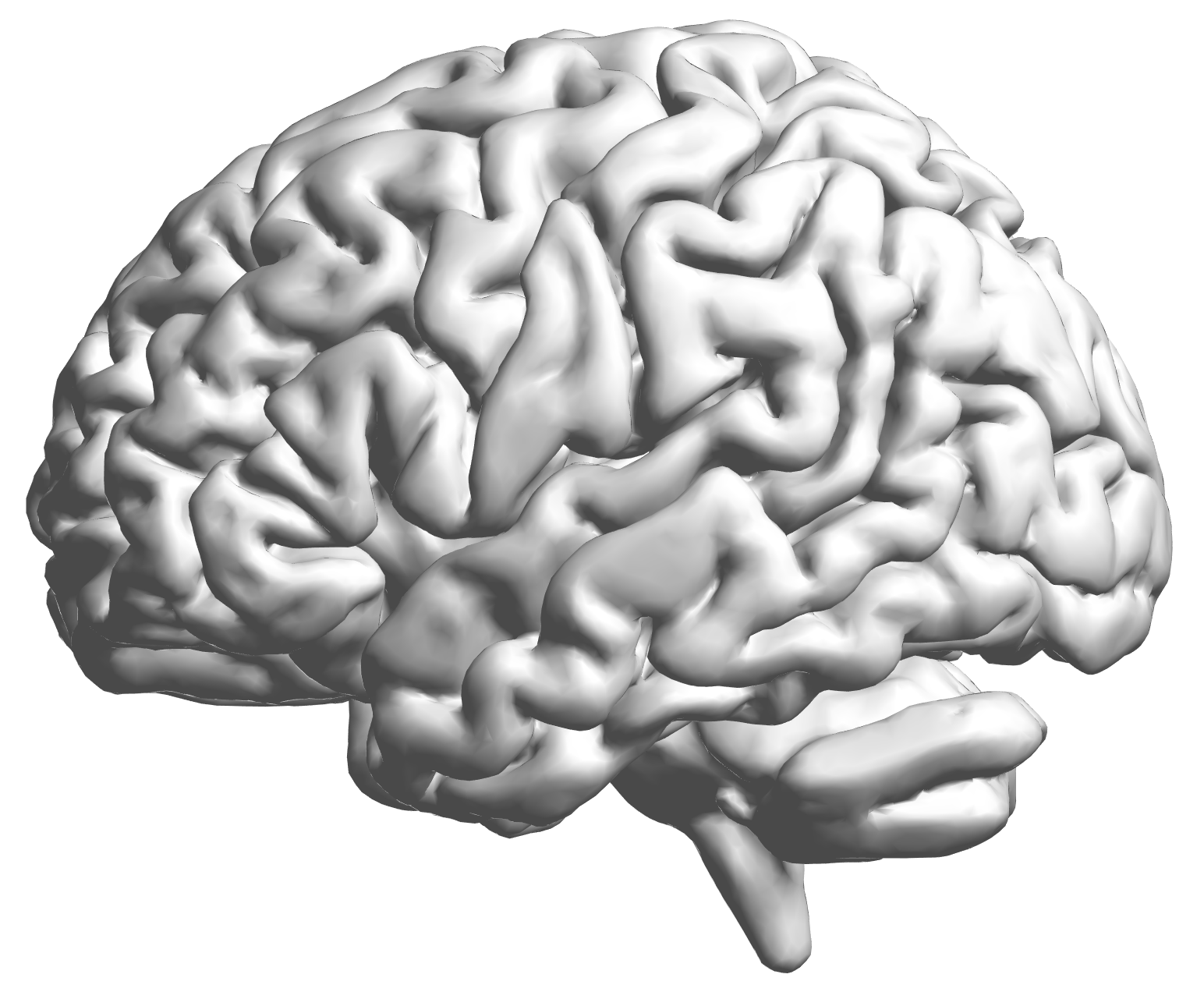• 100 million ( $10^{11}$) neurons
• 100 trillion ( $10^{14}$) neuronal connectionsfMRI data: blood-oxygen-level dependent (BOLD) signals from each cube/voxel (~millimeters), $10^5$ ~ $10^6$ voxels in total.

## Complex Data: fMRI Studies

Sub 1, Sess 1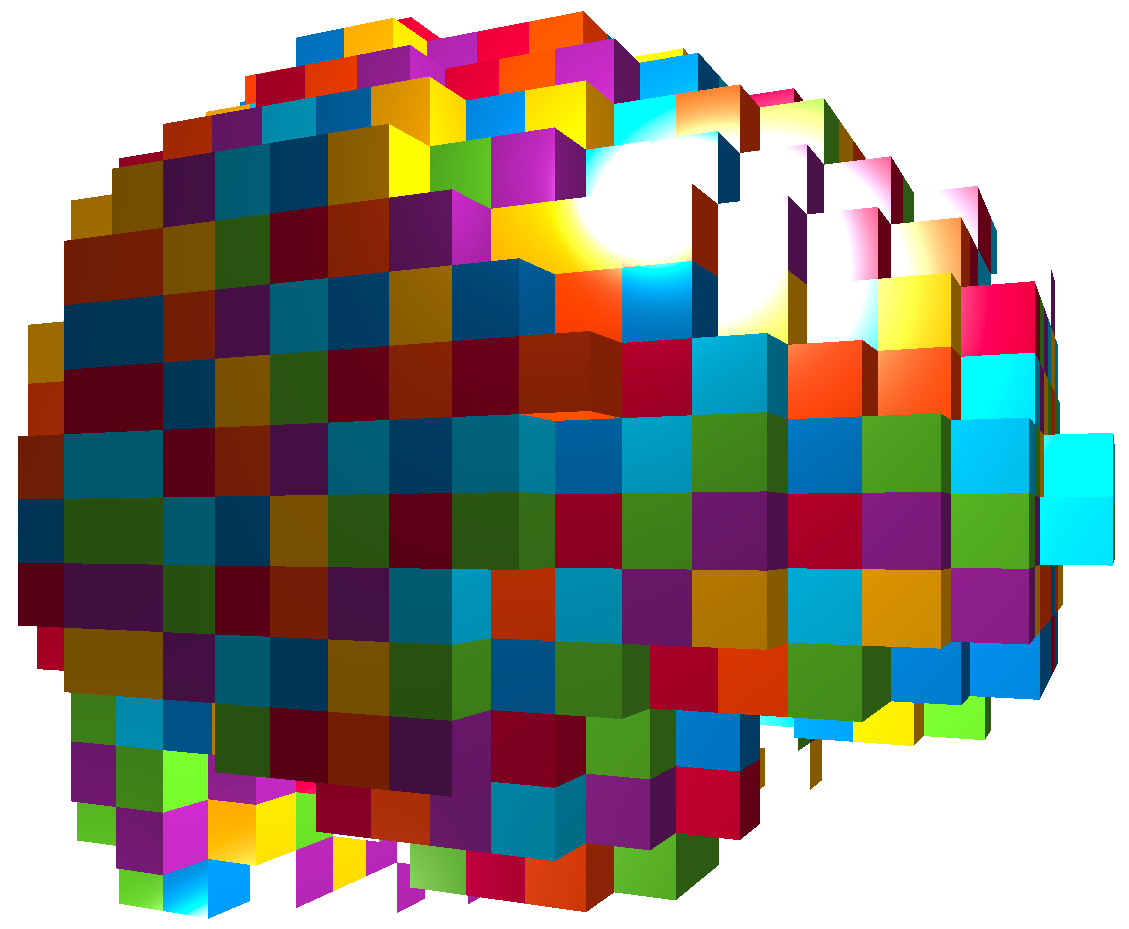Time 12~200

Sub i, Sess jSub ~100, Sess ~4Large, multilevel (subject, sess, voxel) data
$100 \times 4 \times 300 \times 10^6 \approx 100$ billion data points

## Predicting Cocaine Relapses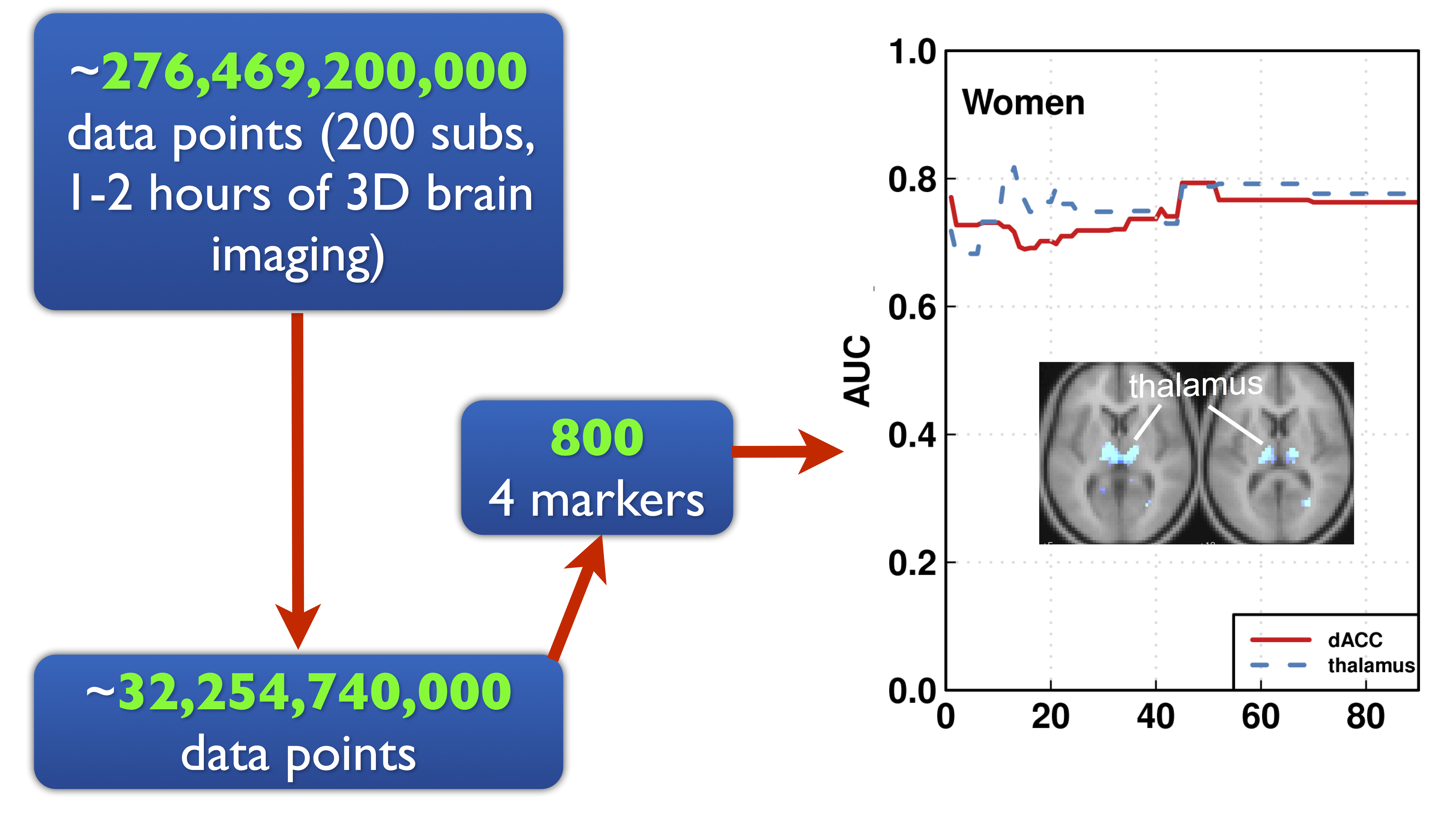Image: Luo et al, 13

## Complex Models: Brain Networks

Find brain networks with 1 trillion ( $10^{12}$) possible connections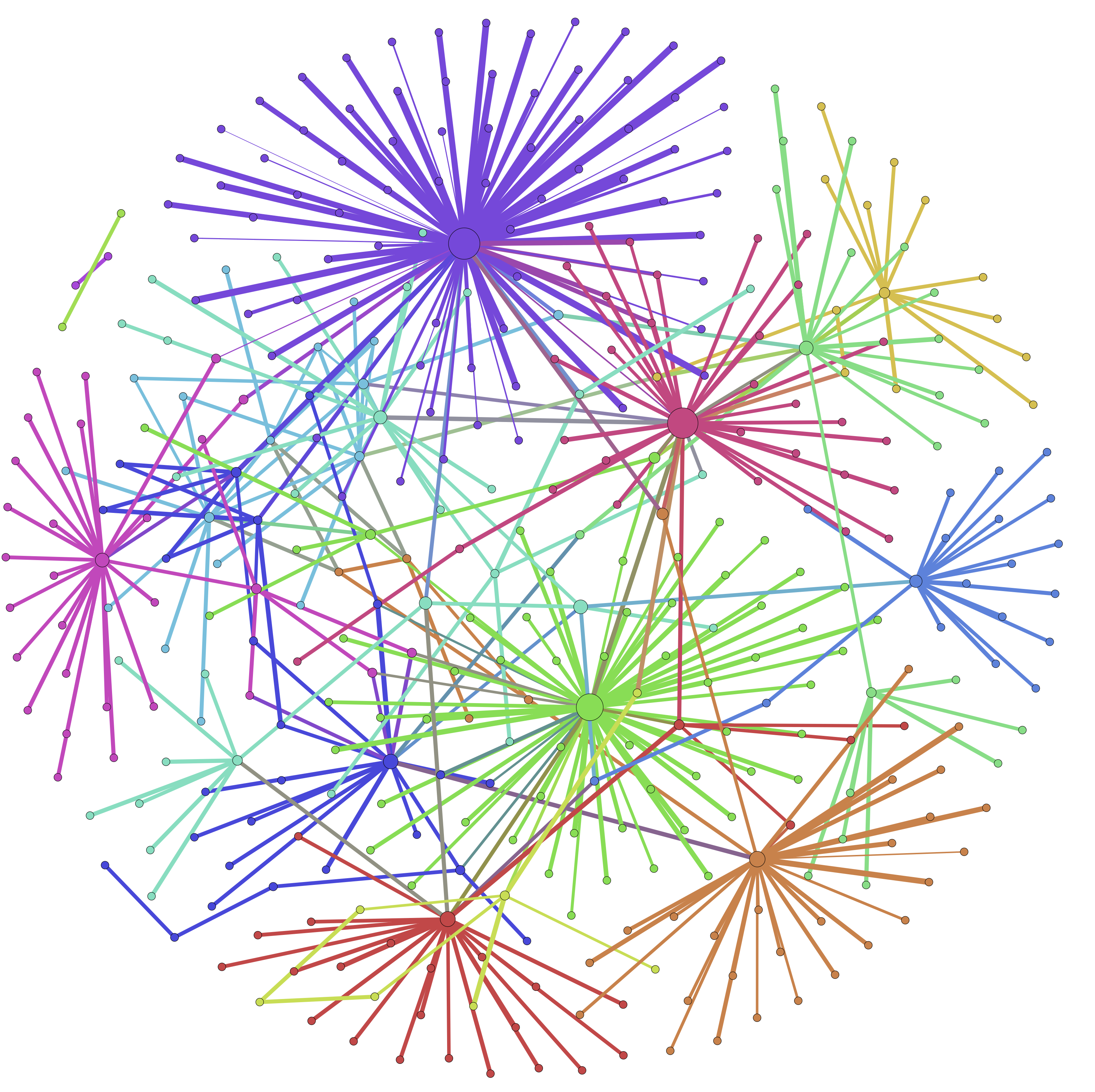Image: Liu and Luo, 15

## Course: Stat Methods for Big Data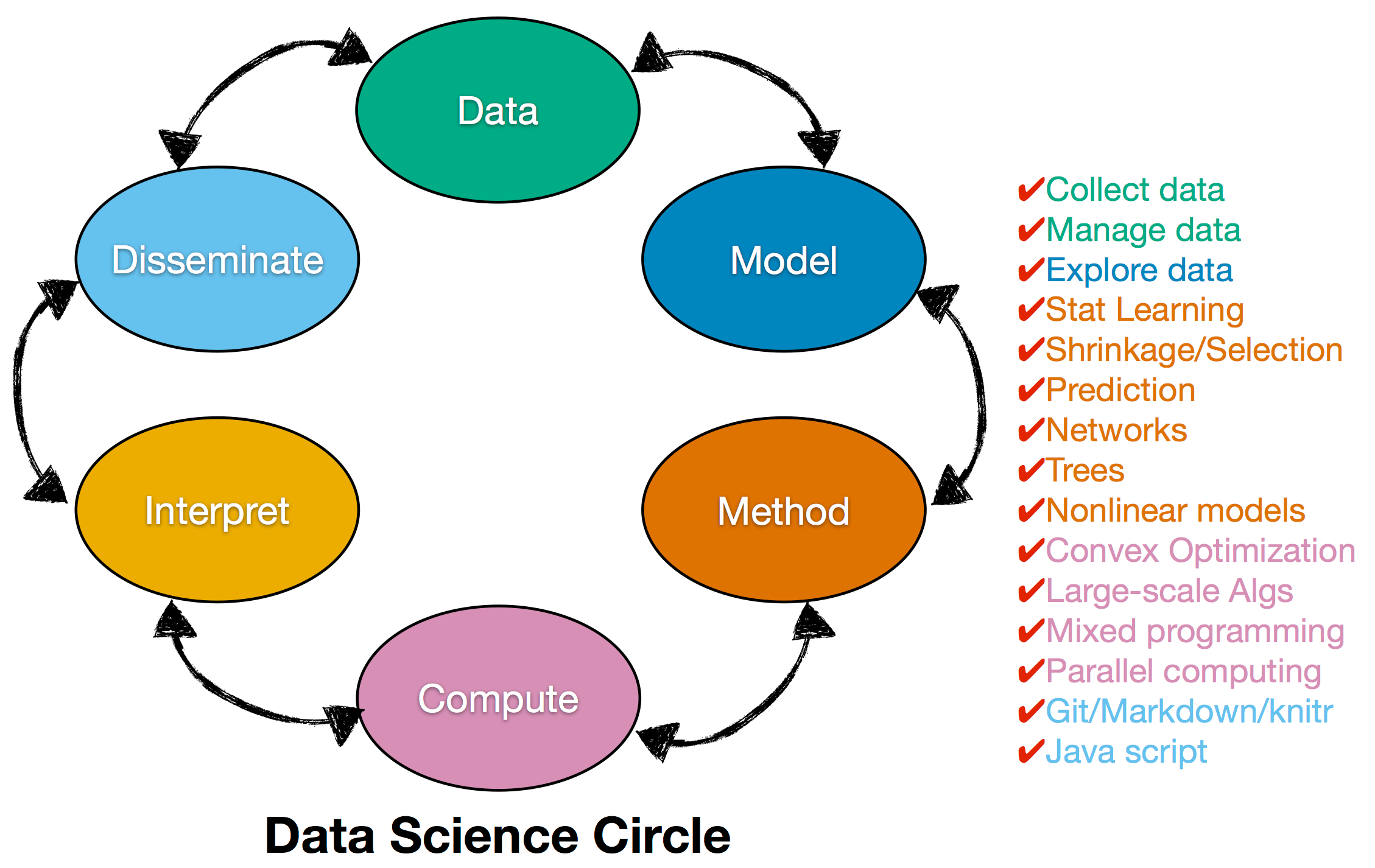Applied Mathematics + Computer Science + Statistics

Thanks to the support of Office of the Provost, the Sheridan Center, and School of Professional StudeisHillary ClintonBernie Sanders

Imge: wikipedia

## Excerpt of DataJaime Zhang, student in SMBDStatistical test: $p<10^{-4}$

Jaime Zhang, student in SMBD

## Summary

• Handle big data
• Complex mathematical modeling
• Extract knowledge from data
• Course SMBD covers the above points